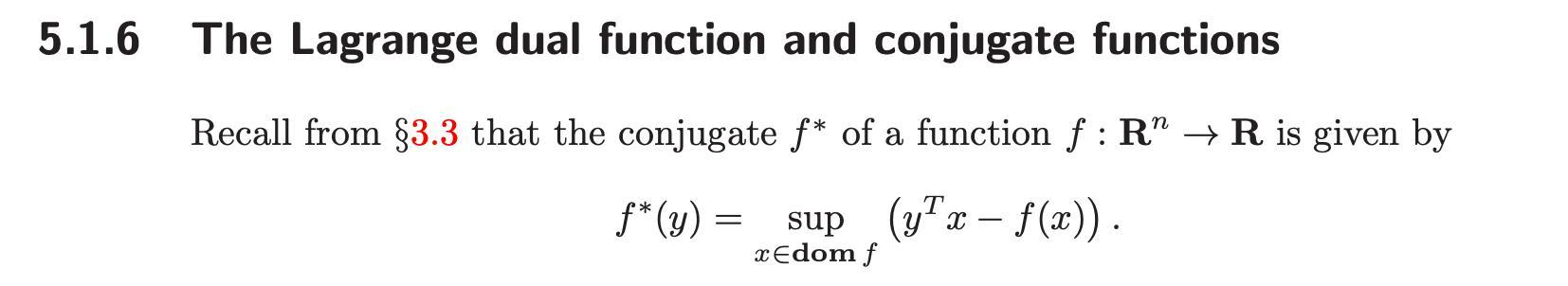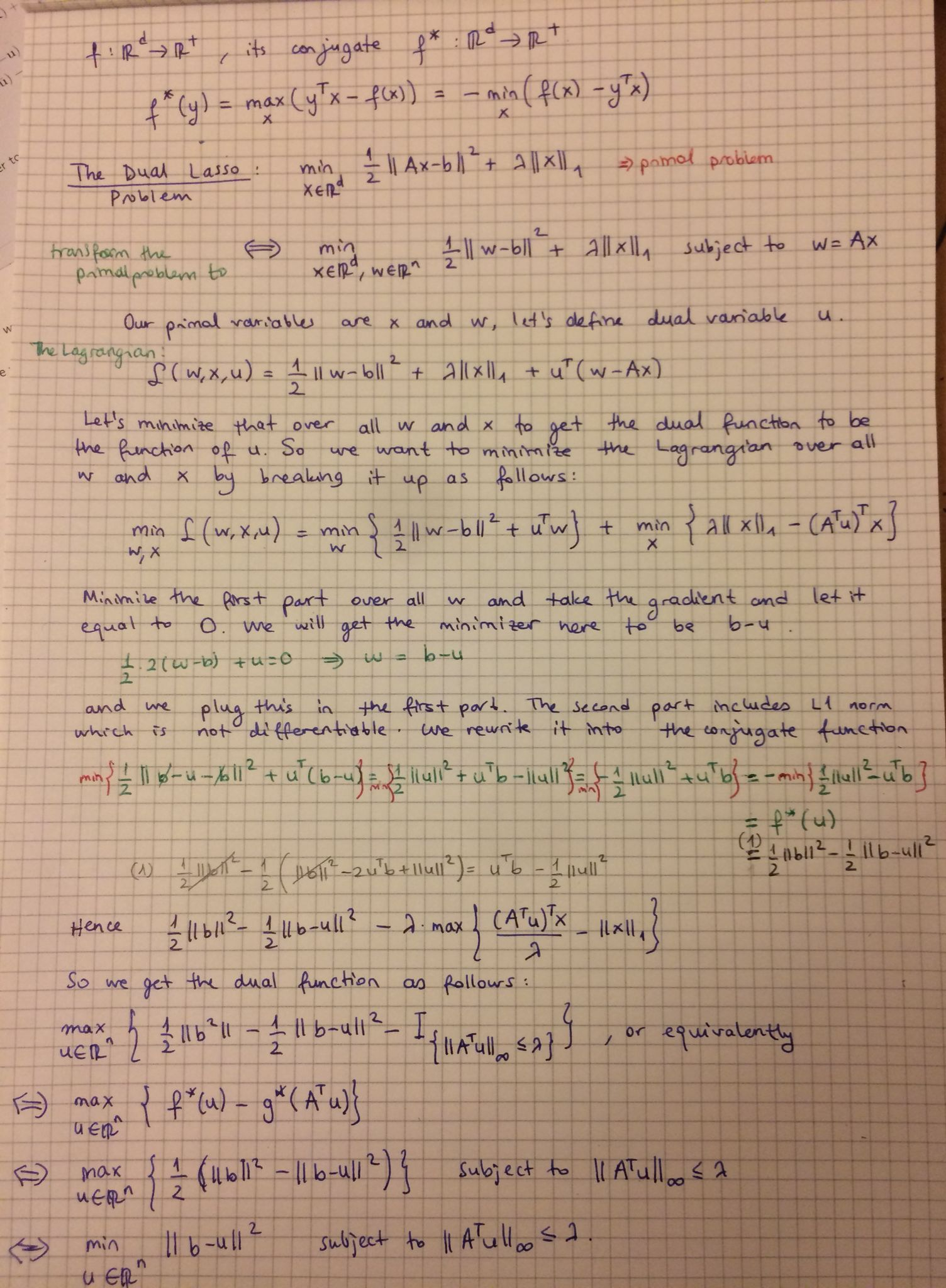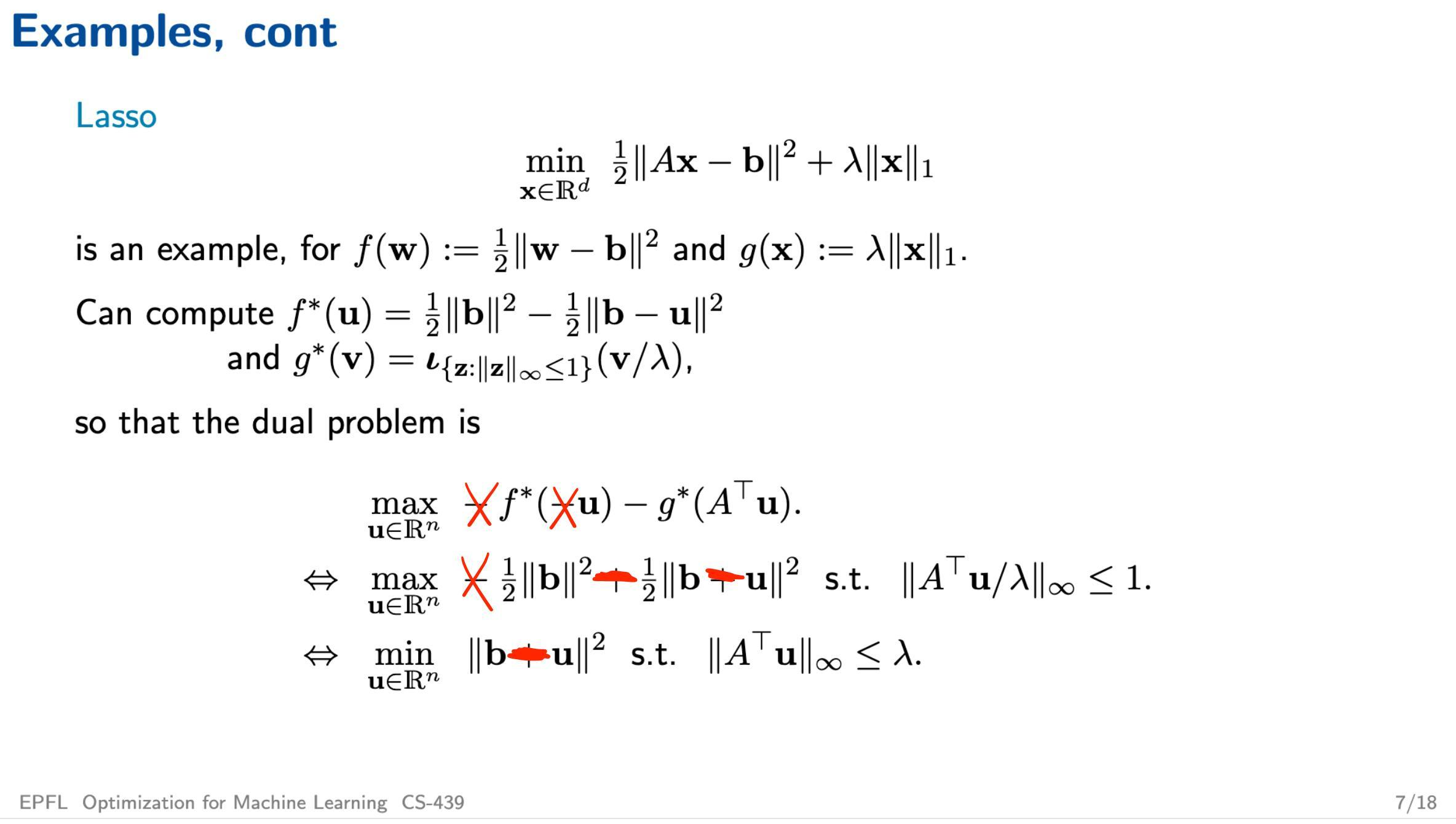### Lecture10 - Duality [Slide 7] - Dual Lasso Problem

Dear CS-439 Team,
In the Q&A session, I remember that with Professor Jaggi, we discussed that the information given on the dual lasso problem might include some typos. However, I couldn't find any changes on the slide or any remarks in the forum based on our discussion, hence I derived the dual lasso as shown in my writing below image.

First thing I realized that I believe that there is a typo in the definition of the conjugate of the function f in the lecture slides. I was confused and checked many sources online because we took $$x^{T}y$$ instead of $$y^{T}x$$. I found out that in B & V cvxbook page 221, section 5.1.6, the conjugate of function f is defined as follow:
Following that, you can find my derivation for the dual lasso problem below. I would be very happy if you could check it and confirm that I actually derived it correctly.Hence, if you also agree that my derivation is correct, I think the slide needs to be corrected like here:Asli

Hi Thijs,

I saw that thread actually, but I don't see why my derivation for the conjugate of the function f is different than yours. But all other dual forms that we both came up with are the same.

Hence, I only have a follow-up question regarding the conjugate of the function f. Could you please check my step for calculating it and tell me what I am doing wrong?

Thanks

Page 1 of 1